Next: Implementation Up: Budan-Fourier method Previous: Budan-Fourier method   Contents

Mathematical background

Budan-Fourier algorithm is a simple method which enable to determine easily some information on the number of root of a given univariate polynomial within a given interval. Let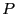the polynomial: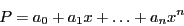and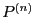its n-th derivative. Let the interval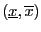the interval in which we are looking for roots. We assume that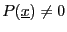,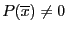and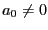. We construct the sequence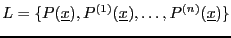from which we exclude the 0 element. Similarly we construct the sequence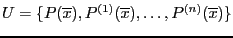(a special treatment has to be applied for the zero element of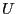, see ). Let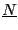the number of change of sign in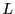and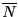the number of change of sign in. Then the number of real roots ofin, counted with their order of multiplicity, is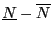or lower than this number by an even number.

Jean-Pierre Merlet 2012-12-20